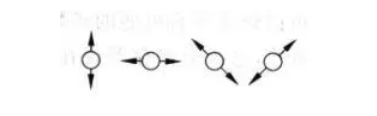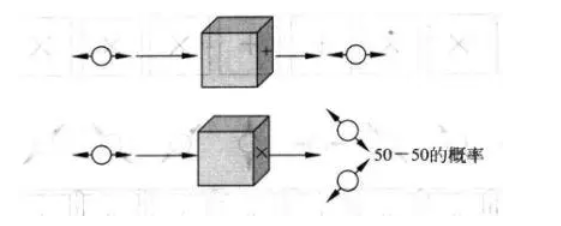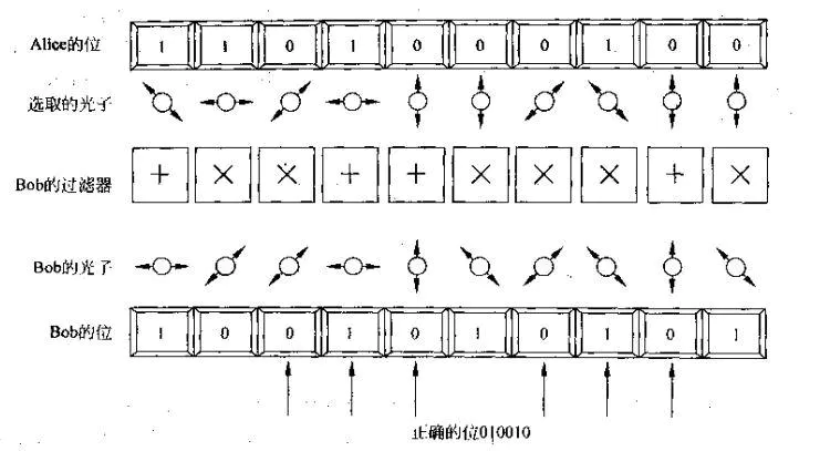# 密码学笔记(二)

## 二、非对称加密

#### 1、RSA

$$72^{139} \mod {253}=2, 105^{139} \mod {253}=101$$
Bob接受到消息（2，101）通过私钥（19，253）解密：
$$2^{19} \mod {253}=72,101^{19} \mod {253}=105$$

$$19e \mod{220}=1$$

#### 2、ElGamal算法

$$c_1=g^{r_1} \mod p = 2^{31} \mod {13171}=4782$$
$$c'_1=m_1 y^{r_1} \mod p = 72×11852^{31} \mod {13171}=7565$$
$$c_2=g^{r_2} \mod p = 2^{16} \mod {13171}=12852$$
$$c'_2=m_2 y^{r_2} \mod p = 105×11852^{16} \mod {13171}=2072$$

$$(c'_1/c^x_1)\mod p=(7565/4782^{23})\mod {13171}=72$$
$$(c'_2/c^x_2)\mod p=(3898/12852^{23})\mod {13171}=105$$

1、找一个较大的素数$p$，例子中是13171；
2、找$g$，它是$Z_p^*$中的生成元，例子中是2；
3、选一个私钥$x$，小于$p-1$的整数，例子中是23；
4、确定公钥$y=g^x \mod p$，例子中是11852。

ElGamal加密法引入了一些新的特征，最显著的是引入随机数，这意味这相同的明文可以有不同的密文，提高了安全性。但是由于密文是明文的两倍大，从而增加了对存储空间的需求，降低数据传输的速度。

#### 3、ECC（Elliptic Curve Cryptography ）

1、Bob先选定一条椭圆曲线，并取椭圆曲线上一点作为基点G。比如$E_{29}(4,20)$，基点$G(13,23)$，基点G的阶数n=37。
$$E_{29}(4,20):y^2=x^3+4x+20 \mod 29$$
G点在椭圆曲线上：
$$13^3+4×13+20 \equiv 23^2 \equiv 7 \mod 29$$

$$37 G =O_{\infty}$$

2、Bob选择一个私钥k（k<n）比如25，并生成公钥K=kG=25G=（14，6）
3、Bob将E、K、G作为公钥传给Alice（全网公布）
4、Alice收到公钥后，将明文编码映射到E上一点，比如是横坐标为“3”，求得纵坐标为28，明文M=（3，28）。再产生一个随机数r，比如r=6
5、Alice对明文加密：
$$C_1=M+rK=M+6K=(3,28)+6\times(14,6)=(6,12)$$
$$C_2=rG=6G=(5,7)$$
（注意这里的加法和乘法不同于我们熟知的加乘法，其规则符合椭圆曲线上的加乘法，详见前文链接）
6、Alice将$C_1,C_2$发给Bob
7、Bob收到密文后，解密明文：
$$M=C_1-kC_2=(6,12)-25\times(5,7)=(3,28)$$

## 三、量子密码学